8 Effective plots with Matplotlib and Pandas Dataframe

In the previous post we learned some matplotlib plotting techniques.This is second part of matplotlib where we are going to work with some random dataset.This post will also cover basic different type of plotting you can produce in matplotlib.This type of plotting are mostly used to understand the type of data and produce useful insights.Learning and understanding matplotlib will take some longer learning time and some patience.While we are trying to learn by creating sample random dataset in dataframe and visualizaling different kind of plots.
I have come to appreciate matplotlib because it is extremely powerful. The library allows you to create almost any visualization you could imagine. Additionally, there is a rich ecosystem of python tools built around it and many of the more advanced visualization tools use matplotlib as the base library.

In :
%matplotlib inline
import pandas as pd
import numpy as np
import matplotlib.pyplot as plt
plt.style.use('ggplot')

The plot method on Series and DataFrame is just a simple wrapper around plt.plot()
If the index consists of dates, it calls gcf().autofmt_xdate() to try to format the x-axis nicely as show in the plot window.

In :
ts = pd.Series(np.random.randn(1000), index=pd.date_range('1/1/2000', periods=1000))

Out:

2000-01-01   -0.383130
2000-01-02    1.122075
2000-01-03    0.264544
2000-01-04    0.205977
2000-01-05    0.652250
Freq: D, dtype: float64

This dataframe consist of data containing random date with random 1000 data points.I have clearly shown the top 5 data by calling head(5) of the dataset.Now, We are going to plot on cumulative sum based on years. This has been shown in below Line plot visualization.

In :
ts = ts.cumsum()
ts.plot()
plt.show()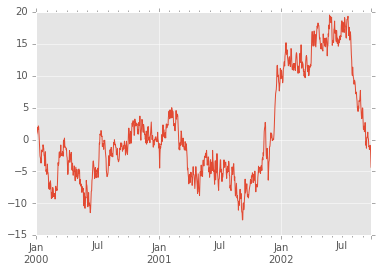On DataFrame, plot() is a convenience to plot all of the columns, and include a legend within the plot.

In the next example we are going to plot multiple plots which will give fair idea about data.This dataframe also contains data for time with random values in 4 marked cloumns A,B,C,D. We are going to see the trends on value in the random generated data.

In :
df = pd.DataFrame(np.random.randn(1000, 4), index=pd.date_range('1/1/2016', periods=1000), columns=list('ABCD'))

Out:

A B C D
2016-01-01 0.734441 -0.967202 1.941327 -0.848996
2016-01-02 1.702695 0.071849 0.668847 -0.751232
2016-01-03 -2.273635 0.385259 -1.347990 0.087448
2016-01-04 0.202025 -1.137845 -0.893557 -0.744962
2016-01-05 -0.094856 -0.090228 0.843362 0.447179

In :
df = df.cumsum()
plt.figure()
df.plot()
plt.show()

<matplotlib.figure.Figure at 0xa34bb70>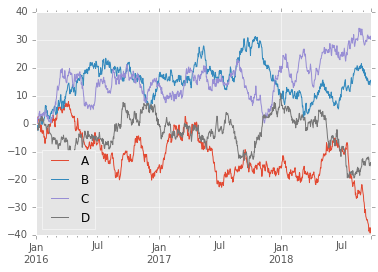Futher we can ses in the above visuallixation which shows a trends using line plot.We are generating cumulative sum for all the columns seperately and plotting it.Based on deomanstrated visualization A columns has produced higher values than others.

In the demonstration ,You can plot one column versus another using the x and y keywords in plot():

In :
df3 = pd.DataFrame(np.random.randn(1000, 2), columns=['B', 'C']).cumsum()
df3['A'] = pd.Series(list(range(len(df))))
df3.plot(x='A', y='B')
plt.show()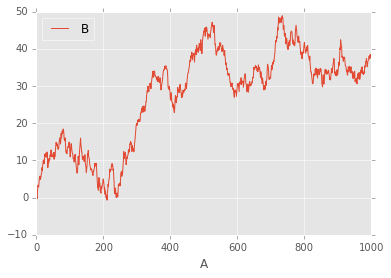In :
df3.tail()

Out:

B C A
995 38.306553 55.991689 995
996 38.052156 55.195136 996
997 38.501586 53.555756 997
998 37.491278 51.671606 998
999 38.449854 50.618397 999

Plots other than line plots¶

Plotting methods allow for a handful of plot styles other than the default Line plot. These methods can be provided as the kind keyword argument to plot(). These include:
• ‘bar’ or ‘barh’ for bar plots
• ‘hist’ for histogram
• ‘box’ for boxplot
• ‘kde’ or 'density' for density plots
• ‘area’ for area plots
• ‘scatter’ for scatter plots
• ‘hexbin’ for hexagonal bin plots
• ‘pie’ for pie plots
For example, a bar plot can be created the following way:.we are going to use the same dataset used above to plot the line plot. I have shown the top 5 column from the dataframe

In :

Out:

A B C D
2016-01-01 0.734441 -0.967202 1.941327 -0.848996
2016-01-02 2.437136 -0.895354 2.610174 -1.600229
2016-01-03 0.163501 -0.510094 1.262183 -1.512781
2016-01-04 0.365526 -1.647939 0.368626 -2.257742
2016-01-05 0.270669 -1.738168 1.211989 -1.810563
2016-01-06 1.906568 -2.422695 2.048194 -2.288180

To plot a bar plot we are fetching index for date 2016-01-06 00:00:00 from dataset and plotting based on the values.
.ix is the most general indexer and will support any of the inputs in .loc and .iloc. .ix also supports floating point label schemes. .ix is exceptionally useful when dealing with mixed positional and label based hierachical indexes.

In :
plt.figure()
df.ix.plot(kind='bar')
plt.axhline(0, color='k')
plt.show()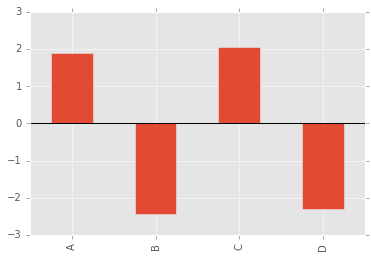In :
df.ix

Out:

A    1.906568
B   -2.422695
C    2.048194
D   -2.288180
Name: 2016-01-06 00:00:00, dtype: float64

stack bar chart¶

Ahhh!.. we are moving to next building a stcked bar chart. we will be creating a small dataset for this demonstration.we will call bar() with stacked=true to plot vertical a stacked bar and we are going to plot a horizontal plot in the next example.

In :
df2 = pd.DataFrame(np.random.rand(10, 4), columns=['a', 'b', 'c', 'd'])
df2.plot.bar(stacked=True)
plt.show()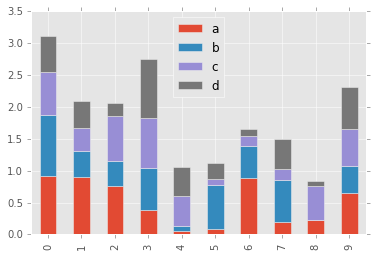horizontal bar chart¶

In :
df2.plot.barh(stacked=True)
plt.show()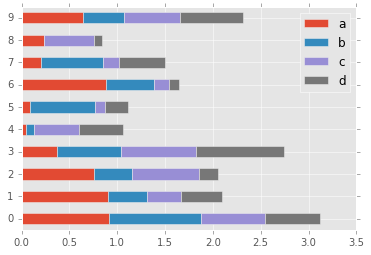Box plot¶

Make a box plot from DataFrame column optionally grouped by some columns or other inputs

In :
df = pd.DataFrame(np.random.rand(10, 5), columns=['A', 'B', 'C', 'D', 'E'])
df.plot.box()
plt.show()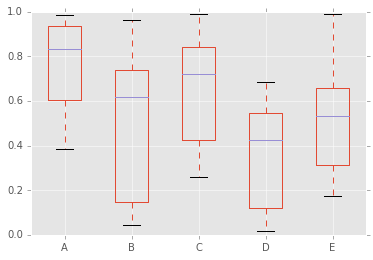area plot¶

In a stacked area plot, the values on the y axis are accumulated at each x position and the area between the resulting values is then filled.

In :
df = pd.DataFrame(np.random.rand(10, 4), columns=['a', 'b', 'c', 'd'])
df.plot.area()
plt.show()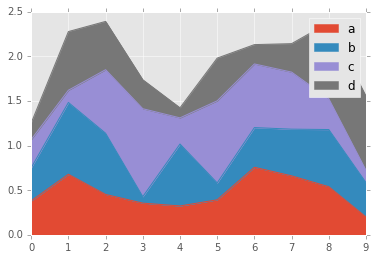Plotting with Missing Data¶

Pandas tries to be pragmatic about plotting DataFrames or Series that contain missing data. Missing values are dropped, left out, or filled depending on the plot type.
Plot Type NaN Handling
Line Leave gaps at NaNs
Line (stacked) Fill 0’s
Bar Fill 0’s
Scatter Drop NaNs
Histogram Drop NaNs (column-wise)
Box Drop NaNs (column-wise)
Area Fill 0’s
KDE Drop NaNs (column-wise)
Hexbin Drop NaNs
Pie Fill 0’s
If any of these defaults are not what you want, or if you want to be explicit about how missing values are handled, consider using fillna() or dropna() before plotting.

density plot¶

In :
ser = pd.Series(np.random.randn(1000))
ser.plot.kde()
plt.show()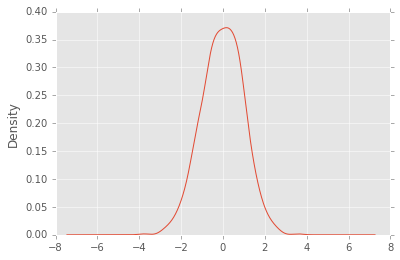lag plot¶

Lag plots are used to check if a data set or time series is random. Random data should not exhibit any structure in the lag plot. Non-random structure implies that the underlying data are not random.

In :
from pandas.tools.plotting import lag_plot
plt.figure()
data = pd.Series(0.1 * np.random.rand(1000) + 0.9 * np.sin(np.linspace(-99 * np.pi, 99 * np.pi, num=1000)))
lag_plot(data)
plt.show()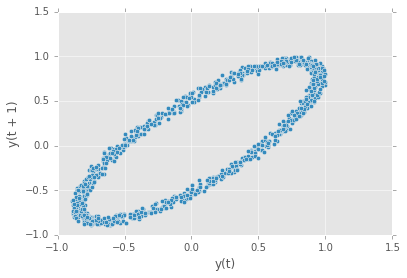PIE Chart¶

In :
# Data to plot
labels = 'Python', 'C++', 'Ruby', 'Java'
sizes = [215, 130, 245, 210]
colors = ['gold', 'yellowgreen', 'lightcoral', 'lightskyblue']
explode = (0.1, 0, 0, 0)  # explode 1st slice

# Plot
plt.pie(sizes, explode=explode, labels=labels, colors=colors,

plt.axis('equal')
plt.show()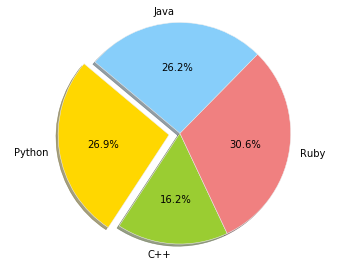matplotlib gallery.¶

documentation: http://matplotlib.org/gallery.html

In [ ]:

Alll the world is a laboratory to the inquiring mind! Join Us